﻿ Differences in Problem Solving Capabilities among Students Given a Problem-Based Learning Blended Learning with Conventional LearningPublications are Open
Access in this journal
Article Versions
Export Article
• Normal Style
• MLA Style
• APA Style
• Chicago Style
Research Article
Open Access Peer-reviewed

### Differences in Problem Solving Capabilities among Students Given a Problem-Based Learning Blended Learning with Conventional Learning

Efiyanti Siregar , Mulyono, Asmin, Mukhtar, Muliawan Firdaus
American Journal of Educational Research. 2019, 7(11), 755-763. DOI: 10.12691/education-7-11-3
Received September 12, 2019; Revised October 27, 2019; Accepted November 07, 2019

### Abstract

There are five mathematical skills that students must have in one of them is problem-solving. The implementation of problem-based blended learning is expected to demonstrate differences in problem-solving skills that are given problem-based learning blended learning and students who are given conventional learning. It is also known that there are interactions between the initial mathematical ability (KAM) and the learning model of problem-solving capabilities so that this is the purpose of this research. The research was conducted in the private junior high school Imelda Medan with the total population of students in the junior high school. And the research samples amounted to 60 students consisting of two classes, the experimental class and the control class. The results showed that there was a difference in problem-solving skills between students who were given learning-based blended learning and students who were given conventional learning. The student problem-solving capability in the experimental class has a higher average of 78,53, compared to an average control class of 71,56. Then there is the KAM interaction and learning model of problem-solving capability with Fhitung value of 3,766 and its significance of 0,029 < 0,05.

### 1. Introduction

Education is one of the ways of establishing human abilities to use the optimal sense and logic as the answer to the problems that arise in the effort to create a good future. According to 1 education is human needs throughout life, without education human thought will be difficult to develop. Therefore, Education plays an important role in life.

This is in line with the opinions expressed by 2 who say that education is a key element of human resource development. Thus, education aims to improve the quality of human resources that have potential in various areas of life in the face of the rapid global competition in the international arena or abroad.

Mathematics is one of the basic science of study that supports the development of science and technology so that mathematics becomes a very important subject in the education level. 1 It says that mathematics is one component of a series of subjects that have an important role in education. As is the case presented by 3 mathematics is a subject studied at a formal level of education ranging from kindergarten, elementary school, junior high school, and high school, and even colleges are not Can be separated from mathematics.

Mathematics as one of the subjects that are expected to contribute to educating students, namely by way of developing basic students ' abilities. Most of the materials in mathematics are closely related, so the basic mathematical mastery is crucial for students to be easier to confront the next material on a more elaborate level.

Soedjadi in 4 stated that one characteristic of mathematics itself is to have an abstract study object that causes students to become lazy to learn mathematics, so that many learning materials cannot be mastered Well, then it is not uncommon to appear complaints that mathematics just makes students confused and regarded as a frightening specter by some students.

The other problems that arise are the learning process that takes place in the classroom is still too dominated by the role of teacher-centered. Teachers play an active role in learning and students are passive to cause saturation and have no good understanding of the material being delivered 5.

There are five general purposes of mathematics lessons formulated by 6 namely: (1) Learning to communicate (mathematical communication); (2) Study for mathematical reasoning; (3) Learn to solve the problem (mathematical problem solving); Learn to associate an idea (mathematical connection); Forming a positive attitude towards mathematics (positive attitudes toward mathematics). All of these educational objectives are the first step to designing good learning to be able to be implemented in the educational world so that the learning objectives can be achieved and produce resources that will be able to become competent With the outside world.

7 stating that low mathematics learning is not only due to difficult mathematics, but it is also caused by several factors that include students themselves, teachers, learning approaches, and learning environments that are mutually related to each other. This is in line with the statement given by the teacher of class IX-A in the SMP Imelda Medan that the results of students learning mathematics are still relatively low. It is said to be low because almost 60% of the mathematical learning outcomes obtained by students are below the KKM value prescribed by the school. The value of the KKM for mathematics lessons at Imelda Medan Junior High School is 70. So to reach the KKM limit value, students still have to go through the remedial stage.

One of the standard processes that students must master is the ability to solve various mathematical problems that are often referred to as problem-solving skills. Student problem-solving skills are very necessary to improve because with the ability of the students can do the organization thinking math either orally or writing, students can respond appropriately, both among students themselves or between students and teachers during the learning process 8. 9, problem-solving is a process that requires logic to find a solution to a problem.

This is following the opinions expressed by Shadiq in 10 namely that problem solving is a very decisive success of mathematics education so that integrating problem-solving during the learning process takes place Must be a priority. So low this ability will result in low quality of human resources, which is demonstrated in low problem-solving capabilities. This is because there is less learning to allow students to develop their skills in solving problems.

According to 10 students who are trained with problem-solving skills will skillfully select important and relevant information, then analyze it. So, in the end, students will conclude. This means that problem-solving capabilities play a crucial role in developing students' thinking potential. But from the research done by 11, in fact math learning tends to have not given any particular attention to the students' problem solving skills, only a small fraction of class VII students are making problem-solving plans on When answering math problems and some students are also difficult to solve the problem that is not routine. In line with the reality in the field, students do not have problem-solving skills, students often do not understand the true meaning of the problem given by the teacher.

Related to the importance of problem-solving skills in mathematics learning that is not in line with the reality in the field is also seen in students in class IX Middle school Imelda Medan which shows that problem-solving skills are still relatively low. This is known from the results of preliminary studies conducted by researchers in class IX of SMP Imelda Medan by looking at the process of students ' answers are still rigid and not able to show how the complete steps of the problem given. Even the student can not determine the mathematical model that is in question relating to problem-solving skills. So it can be concluded that the ability to discharge problems in grade IX SMP Imelda Medan still needs to be improved.

One of the factors that resulted in low student problem-solving skills is the learning process that is applied in the school SMP Imelda Medan still use conventional or direct learning or regular learning that is one direction.

Conventional learning is a learning process that is fully controlled by the teacher because in this learning the teacher plays a dominant role which emphasizes more of the delivery of new information by the teacher to the students. In this case, students are seen as objects that receive what teachers are given.

One of the steps teachers can take to improve students ' problem-solving skills is to choose a model that is precise and nuanced to the competency of the students, especially the ability to solve Active in the learning of mathematics.

The learning model required for students' problem-solving skills to thrive is to use a model that makes learning meaningful. Meaningful learning in mathematics is learning where students can find their own needed knowledge, what skills are used and ultimately able to solve the problems given. One example is the blended learning model.

Blended Learning Learning is a combination of traditional learning and an electronic learning environment. Blended learning lessons incorporate web/Internet-based learning aspects, video streaming, Synchronous audio communication and asynchronous with traditional or face-to-face learning. 12 also said that the learning of blended learning is a process of unifying various learning methods that can be accomplished by merging virtual and physical sources. Thus, blended learning is essentially a combination of face to face learning and virtual (e-learning) excellence.

The advantages of this learning model include (1) Flexibility, meaning that students can contribute to the discussion at the time and place they choose individually. (2) Participation means that all students can participate in the learning process because they can arrange the time and place to participate. (3) Learning has more time so that it can be more careful in arguing and more in reflecting its views and opinions.

To implement a blended learning model more meaningful, learning is needed to facilitate the students in that direction. Problem-based learning is considered capable to direct students to a meaningful learning process.

Based on the results of the study conducted by 13 which concluded that students taught with problem-based learning achieved better average grades and increased by 43% compared to students studying Conventionally.

Problem-based learning is learning where students work with authentic issues with the intent to compose their knowledge, develop skills and self-reliance and be able to improve confidence. Problem-based learning measures are orienting students on issues, organizing students to learn, guiding individual experiences/groups, developing and presenting work, and analyzing and evaluating processes Troubleshooting. The phases in problem-based learning provide students with opportunities to improve student problem-solving skills.

This is in line with Selcuk's opinion in 14 which says that problem-based learning makes students more active in learning so that they can improve student problem-solving skills.

In addition to student problem-solving skills, students' success in math learning is also heavily influenced by the early mathematical abilities (KAM). This early mathematical ability is decisive in learning a new mathematical subject matter because mathematics has a hierarchical nature. The better the student's early math skills will be the better the ability for students to learn the math material that will be learned. Besides, the initial ability of student mathematics is also useful as a foothold in the optimal selection of learning strategies. Because by knowing the early math skills of each student, teachers will be younger in determining methods or strategies suitable for use in the classroom so that the learning performed will be more effective and efficient.

Early mathematics skills at SMP Swasta Imelda Medan are still relatively low. This is evident from the results of the students ' responses at the time when researchers gave the students an initial test of math skills related to quadratic equations in class IX A and IX B. Which results show that only 20% of the total number of students capable of answering the test in the experimental class and 13% in the control class with the highest score of 90 in the experiment class and 87 in the control class. So the researchers concluded that the initial ability of mathematics (KAM) students in SMP Swasta Imelda Medan is still relatively low.

Thus, the implementation of a problem-based blended learning model is expected to enable students to follow learning. From the exposure of the problems and facts that have been submitted, researchers conducted a study to see the difference in problem-solving skills among students who were given problem-based learning blended and conventional learning.

### 2. Research Methodology

This research was conducted at SMP Swasta Imelda Medan on the odd semester of lesson 2019/2020. This research is pseudo experimental research (quasi-experiment) because the research wants to know and analyze the difference of treatment with other treatment. The population in this study was SMP Imelda's year's lesson 2019/2020. The sample withdrawal technique used is a simple random sampling. This is done for class equality to be measured. So the researchers took a class of IX A, and the IX B class, the two classes chosen were selected randomly to be used as experimental classes that would be given a problem-based blended learning study treatment and the other class was made Class with conventional learning.

This type of research is a quasi-experiment (a pseudo experiment) because the class used has been formed previously. The free variables in this study are the problem based on blended learning and conventional learning. And bound variables are students' problem-solving skills after being given a problem-based blended learning and conventional learning treatment.

The instrument used in this research is a test of the problem-solving skills of students given after treatment to measure the ability of students. This test problem is about 5 questions in the form of troubleshooting. Before the problem is given to students, the question should be tested validity which is determined based on the suitability of the grating problem with the grain.

Before the instrument is tested, the validation of the instrument is done by some experts. Furthermore, the test stage of learning devices and research instruments. A test or measuring instrument has a high validity when the test performs its measuring function or provides a measurement that matches the purpose of the measurement itself. Thus to know the validity of the grain problem in this research used the correlation formula product of the moment as follows: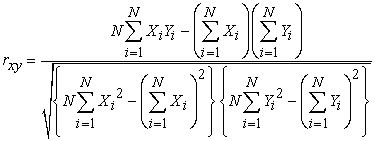Description: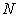: Number of a student taking the test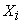: Scores Earned on each item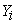: Total scored of each item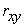: Coefficient of validity test.

Next to know the significant correlation obtained, calculated using Uji-t, with the following formula: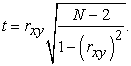The criteria must be fulfilled so that an item is said to be valid if thitung> ttabel with ttabel =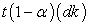for dk = N-2 and α (significant level) is selected 5% The Instrument is valid.

To test the reliability of the form test, the alpha formula is used 15: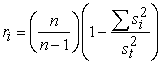Description: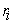: Overall test reliability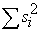: Number of score variances of each problem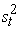: Total Variance

n: Number of items in the test.

Then to calculate the difficulty index about using the difficulty index formula as follows: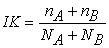Description: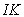: Difficulty index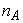: The number of top group students who answered the questions correctly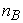: Many lower-class students answer correctly: The number of top group students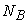: The number of students in the lower classes

Then a question is said to have a good distinguishing power if the clever students can work on the problem well and the less clever students can not answer the question well. Calculation of differentiator power of each problem for description is to use formula as follows: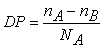Description: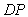: Distinguishing items: The number of top group students who answered the questions correctly: Many lower-class students answer correctly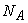: The number of top group students.

The data processing techniques in this study are by using descriptive statistical techniques and inferential statistics. Inferential statistics are statistical techniques used to analyze sample data and the results applied to the population.

Then the analysis used in this research is quantitative analysis (inferential). Quantitative analysis is used to analyze the data obtained from the problem-solving ability scores grouped according to the Maslalah-based blended learning and conventional learning.

Then the analysis used in this research is quantitative analysis (inferential). Quantitative analysis is used to analyze the data obtained from the scores of problem-solving capabilities grouped by problem-based blended learning and conventional learning.

### 3. Results and Discussion

To answer the formulation of problems expressed in the introduction, necessary analysis and interpretation of data from the research results to fulfill the purpose of this research. The main purpose of this research is to analyze the differences in problem-solving skills students are taught using the learning model of blended learning based problems with conventional learning. It is also to find out if there is any interaction between the learning model and the initial mathematical ability of the students ' problem-solving skills.

3.1. Research Result

Data on the results of the study consist of diagnostic test data, test data on student problem-solving.

3.1.1. Description of Beginning Mathematics Ability (KAM) Students

Students' early math skills are measured through the data collected based on the diagnostic tests that have been given to both classes. Results of the average summary and standard deviation of the KAM as shown in Table 2 following:

3.1.2. KAM Data Normality Test

One of the requirements in quantitative analysis is the fulfillment of the data distribution's assumptions that will be analyzed. The normality test used in the study uses the Kolmogorov-Smirnov test with a significant 5% level. The hypotheses tested were:

Ho: Samples derived from the normal distribution population

Ha: Samples derived from a population that is not a normal distribution

The summary result of the KAM data normality test calculation is presented in Table 3 as follows:

From Table 3 above it appears that the value of significance Kolmogorov Smirnov is also 0.112 and 0.060 for experimental classes and control classes. The second significant value of the second class is greater than the significant value of 0.05, so the null hypothesis stating normal distribution data for both classes is acceptable. In other words, the data for both classes have normal distribution data.

3.1.3. Homogeneity Test for KAM Data

After conducting the normality test, testing the homogeneity of the variance of the experimental class and the control class at a significant 5% level. The results of the calculation of the homogeneity test of both classes are presented in Table 4, following:

From Table 4, the above appears that a significant value of 0857 is greater than the significant equivalent of 0.05, resulting in the null hypothesis that states there is no difference in the variance between groups. This indicates that the two data groups of the two classes have homogeneous data variances.

3.1.4. KAM Data Average Similarity Test

The average similarity test was done to see the similarities in the original students ' early math skills from the experimental class and the control class obtained from the diagnostic tests given. These results were analyzed with Uji-t with the following hypothesis: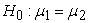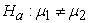Description: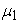: The average initial ability of mathematics (KAM) student class experiments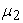: The average initial ability mathematics (KAM) student class controls

The results of calculating the average similarity test from both classes are presented in Table 5.

Based on the calculations in Table 5 using Uji-t level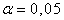retrieved thitung of 0,105 while ttabel of 2,04. Because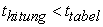is so the null hypothesis is acceptable. This indicates that the two data groups of both classes have the same capabilities.

Furthermore, grouping early students' mathematics skills (high, medium and low) is formed based on the students ' KAM. For students who have KAM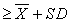be grouped in high ability, while students who have KAM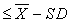be grouped in low ability. For a learning class-based blended learning model with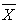= 73,70 and SD = 14,32 so that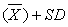= 88,02 and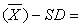59,38. As for the conventional learning class the value= 67,40 and SD = 15,33 so that= 82,73 and52,07. Results of a sample distribution summary presented in Table 6.

Based on Table 6 gained on the class of experimentation levels of students for high category there are 6 students, medium 17 students and low there are 7 students, while the class controls the ability level of students to high category there are 4 students, medium 20 students and low there are 6 students.

3.1.5. Description of Test Results Troubleshooting Abilities

After the learning model has been applied to the experiment class, it is given a problem-solving ability test to determine the extent to which students can solve problems after being given learning. The test results for both classes are described in Table 7.

Based on Table 7, it appears that the minimum value of test problem-solving skills of students in the experiment class is 50, and higher than in the control class whose minimum value is 43. For the maximum value of student problem-solving skills in the experimental class is 95, and higher than in the control class whose maximum value is 90. Thus the average posttest student problem-solving capability for the experimental class was 78,53, higher than that of the control class students whose population was 71,56. The standard deviation test is a problem-solving capability for the experimental class of 14,91, higher than that of the control class students whose deviation is 15,75.

3.1.6. Test Data Normality Test Troubleshooting Capabilities

The output of the test calculation of data normality test student problem-solving ability is presented in Table 8. following:

Based on Table 8, the above appears that the significance values of the test's normality in test data are 0,052 and 0,053 for experimental classes and the control class is greater than the significant 0,05, resulting in the null hypothesis that states normal distribution data for Both classes are acceptable. In other words, the data for both classes have normal distribution data.

3.1.7. Test Data Homogeneity Test Problem-solving Ability

As for the output of the test calculation data homogeneity test students problem-solving skills presented in Table 9, following:

From Table 9 above it appears that the significance value of 0,816 is greater than the significant equivalent of 0,05, resulting in the null hypothesis that states there is no difference in the variance between groups. This indicates that the two data groups of the two classes have homogeneous data variances.

3.1.8. Hypothesis Test

Statistical hypothesis testing was conducted using the two-way ANOVA after the fulfillment of a normal distribution data and a homogeneous variance of data groups.

Based on the results of the problem-solving capability test, the following hypothesis test is conducted:

Research hypothesis 1: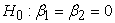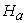: there is at least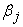one non-zero

Description :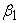: the average problem-solving ability of students taught using the problem-based Blended Learning model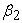: the average problem-solving ability of students taught using conventional learning

Research hypothesis 2: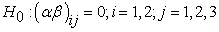: there is at least one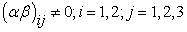Description: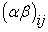is the interaction between a learning model and KAM on student problem-solving skills.

The 1 and 2 hypothesis test results with two-way ANOVA using the SPSS program can be seen in Table 10, following:

Based on Table 10 above it looks that for the early mathematical ability (KAM) acquired value Fhitung of 98,238 and significant value of 0,000, because the value is significantly smaller than 0,05 then it rejected Ho and received Ha. So, KAM's category affects problem-solving ability. It can be seen also that for learning factors with KAM obtained the value Fhitung of 3,766 and significant value of 0,029, because the value is significantly smaller than 0,05 then it rejected Ho and received Ha. Thus, it can be concluded that there are significant interactions between learning models (problem-based and conventional blended learning) with the initial mathematical ability (KAM) on problem-solving skills. It can be interpreted that there is a common influence given by the learning model and the initial mathematical ability to problem-solving skills.

It can be interpreted that there is a common influence given by the learning model and the initial mathematical ability to problem-solving skills.

3.1.9. Test Post Two-Way ANOVA's Advanced Test on Troubleshooting Capabilities

Advanced test post-two-way ANOVA is conducted using the Scheffe method (with the help of SPSS version 21 software calculations) to find out which is higher among the learning group of model interactions with KAM in improving the ability Solving problems in this study. As for the advanced test hypothesis to test student troubleshooting skills as follows:

1.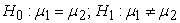2.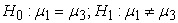3.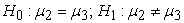Keterangan:: The average problem-solving capability in high mathematical early capability groups: The average problem-solving capability in medium mathematical early capability groups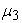: The average problem-solving capability in low mathematical early capability groups

As for the results of the post-two-way ANOVA Advanced test for problem-solving capabilities will be presented in Table 11. Following:

The Scheffe test results (Table 11) can be summarized in Table 12, following:

Based on Table 12, then it can be described testing the two-way ANOVA Advanced test hypothesis on the following troubleshooting capabilities:

1. The learning Model of the problem-based blended learning and conventional a different result if it is performed in children who have a high and low KAM, but not so if given to students who have a moderate KAM. By looking at each average, it can be concluded that the problem-based blended learning model is more effective than conventional learning when given to students with high and low KAM.

2. For students given conventional learning, each different KAM level gets an average of different problem-solving abilities. By looking at the reality, it was concluded that on conventional learning students who had high KAM had better problem-solving capabilities compared to students who had moderate KAM, and students who had the KAM had better ability Solving problems compared to students who have low KAM.

3. For students given learning-based blended learning problems, students who have lower KAM are more ugly problem-solving skills compared to students who have moderate and high KAM.

It can also be described in Figure 1.

In conclusion of research based on Figure 1 (by looking at ANOVA results and further testing) as follows:

1. In general, students who have high KAM have better problem-solving skills compared to students who have moderate KAM, and students who have KAM are better than those who have low KAM.

2. By influence, problem-based blended learning models and conventional learning influence problem-solving skills. But when viewed from the KAM, the implementation of problem-based blended learning and conventional learning for students who have the KAM is going to produce the same problem-solving skills.

View option
• Figure 1. Interaction between learning models and the initial mathematical ability of problem-solving capabilities
3.2. Discussion of Research Results

Based on research findings and data analysis results, it will be seen in conjunction with the research objectives that have been established. The discussion of the research will be outlined based on several factors related to this study, namely the learning model factor and problem-solving ability.

3.2.1. Learning Model Factors

Learning models are one of the factors that affect students' problem-solving skills. Each stage of the learning model contributes to the ability to problem-solving and learning the independence of students.

Each stage of the learning model contributes to the ability to problem-solving and learning the independence of students. The presence of social interactions with peers (peer tutors) or even with their teachers will affect the communication skills of students in groups and other groups and shared responsibilities in the group and equipped with teachers who role as a motivator, facilitator, and moderator will enable more quality discussion and more effective learning.

In each class of researchers initiated learning through the presence of cognitive conflicts, it is in line with the theory of constructivism learning. At the end of the learning process, knowledge will be built by the child through his experience of interaction with his environment. Also, learning prioritizes meaningful learning, the meaningful theoretical theory of Ausubel emphasizes the importance of students associating new experiences, phenomena, and facts into their structure. Both emphasize the importance of assimilation of experience into cognitive structures and the learning process is the way students are active.

Problem-based blended learning is learning that emphasizes on the situation or condition of real problems and related to the daily life of students. With the implementation of the problem-based blended learning issue in learning is expected that students can more easily understand what concepts are actually wanted to be delivered, and can also develop the knowledge of students that have been possessed to be more complex.

3.2.2. Problem-Solving Skill

Based on the results of a descriptive data analysis after being given the treatment, the problem-solving skills of students learning with problem-based blended learning are higher than students who learn with conventional learning.

The results were analyzed with ANOVA two lanes and it can be concluded that there are different problem-solving skills of students who learn through problems based blended learning and conventional learning.

The results of this study in line with 16 titled The Application of Blended learning with the application of Edmodo based Pdeode learning strategy to improve students ' learning performance concluded the application of blended learning with Edmodo application Based on the PDEODE learning strategy can improve the student learning performance of VIIIF MTs N Magelang.

Another study conducted by 17 titled Influence of Blended Learning models assisted animation video on problem solving skills and understanding the concept of students gained the result that understanding the concept of mathematics and solving ability the problem of students who follow the blended learning models of animated videos is better than understanding the mathematical concepts and problem-solving skills of students who follow traditional models.

Reviewed from the research process that researchers gained that students ' problem-solving skills were given better-based blended learning problems than the students ' problem-solving skills given conventional learning. This was seen at the beginning of learning to teach and learning activities in the experimental class faster to get a response from the students compared to the control class.

3.2.3. Interaction between Learning Models with Early Mathematical Ability to Problem-Solving Skills

Based on the results of inferential statistical analysis with two-way ANOVA test on the initial line of mathematical ability * Model obtained its significant value 0,029 < 0,05, it can be concluded that there is an interaction between learning models (blended learning Problem-based and conventional learning) with the initial mathematical ability to solve students' problem-solving skills.

The interaction between the learning model and the initial mathematical ability of students ' problem-solving skills means that, together with the learning model and early mathematical skills, the students' problem-solving skills of both classes, the experimental class, and the control class. With the influence of interaction, it cannot be concluded directly that one of the learning models is better than others. Further testing is required for students ' troubleshooting data.

From the results of the advanced test, there is a difference between the two average students' problem-solving skills from both learnings. This is due to differences in the learning stages of both learning models. A problem-based blended learning model will be attracted by the problem-solving process so that the understanding will last longer, while in conventional learning students rely more on the teacher to obtain information.

### 4. Conclusion

Based on the analysis of research data and research discussion of the learning model of blended learning based problems and conventional learning to problem-solving skills in class IX SMP Swasta Imelda Medan, then researchers acquire some conclusion that is the answer to the questions posed in the problem formulation. The conclusion is as follows:

1. There are differences in problem-solving skills of students who are given learning-based blended learning problems with students who are given conventional learning.

2. There is an interaction between the model (problem-based blended learning and conventional learning) judging from the early ability of mathematics to students' problem-solving skills. It also means that the interaction between learning models (problem-based blended learning and conventional learning) and initial mathematical ability (high, moderate and low) has been put into effect together with the ability problem solving of the student is due to the learning differences used and also because of the student's early mathematical skills.

### Acknowledgments

In the implementation of this research, the author got some help, guidance, and direction of various parties. Therefore, on this occasion the author would like to extend a sincere thank you to the most important God Almighty, my Father loved both parents, Alm. Nazaruddin Siregar and Mama dearest, Lanna Harahap, a character who fought raising and always pray for all at once became my inspiration, my beloved brother and sisters, Ratna Dewi Siregar (Dewi), Mery Romaito Siregar (Mei), Ahmad Husein Siregar (Husen), which always gives love and teach the meaning of brotherhood and mutual reminding and support to continue reaching your goals and success, the SMP Swasta Imelda Medan that has allowed me to do some research and obtain the necessary data in this study.

### References

Published with license by Science and Education Publishing, Copyright © 2019 Efiyanti Siregar, Mulyono, Asmin, Mukhtar and Muliawan FirdausThis work is licensed under a Creative Commons Attribution 4.0 International License. To view a copy of this license, visit http://creativecommons.org/licenses/by/4.0/